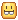# How to make a part be in a random location

for e.g.all the coins is in the circle + its random
that’s what I wantthis is what I don’t want even though it’s random
its still not in the circle

I don’t know the word for it but i know what it is
(no responses)

3 Likes

have a vector for the origin point in the middle of the circle,
you want 4 vectors to create a square in each corner of the desired range (offset from your origin)
local pos = math.random(minX,maxX,YPos,minZ,minX)

after pos is gotten you want to magnitude check to see if its distance fits the criteria for your circle area e.g you want a 30 stud circle, if its outside of the range then repeat until desired output

1 Like
``````local function getCoord(centre, max) -- Centre is not Vector3, just an axis
local plusMinus = math.random(1, 2)

if plusMinus == 1 then
return centre  + (max * math.random()) -- Gets a random percentage of the max, i.e. 50% of the max
else
return centre - (max * math.random())
end
end

local function getSpawnPos(centrePos, maxX, maxY, maxZ) -- Centre of the coin spawn, then how far it can spawn away on each axis
local x = getCoord(centrePos.X, maxX)
local y = getCoord(centrePos.Y, maxY)
local z = getCoord(centrePos.Z, maxZ)

return Vector3.new(x, y, z)
end
``````

Example:

``````local coin = ...
coin.Position = getSpawnPos(Vector3.new(0, 0, 0), 10, 15, 10)
``````
1 Like

Assuming your boundary is a perfect circle, you can generate two values, one being the angle (0 <= angle <= 360), another being the offset (where 0 <= offset <= circle’s radius) to obtain a random position within your circular boundary. Something similar to Polar Coordinate System.

1 Like
``````local randomObject = Random.new(tick())

local storage = game:GetService("ServerStorage")
local coin = storage.Coin

local function getRandom(value)
return randomObject:NextInteger(-value, value)
end

local function randomCoin(origin, offset)
local coinClone = coin:Clone()
coin.Position = origin + Vector3.new(getRandom(offset), origin.X, getRandom(offset))
coin.Parent = workspace
end

randomCoin(Vector3.new(0, 0, 0), 5) --Creates a randomly positioned coin around the origin point (0, 0, 0) with an offset along the X-axis and Z-axis between the range of -5 to +5 studs from the origin.
``````

I’ve added comments which should help explain the script (it’s just an example).

1 Like